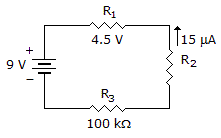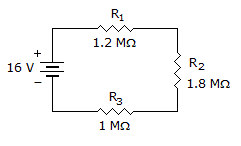# Electronics - Series Circuits

### Exercise :: Series Circuits - Filling the Blanks

1.

The ______ determines whether connected resistors are in series, parallel, or series-parallel?

 A. current flow B. power source C. voltage flow D. wattage source

Explanation:

No answer description available for this question. Let us discuss.

2.

If a 3.9 k, a 7.5 k, and a 5.6 kresistor are connected in series with a 34 V source, the voltage dropped across the 7.5 kresistor equals _____.

 A. 34 V B. 15 V C. 7.8 V D. 11.2 V

Explanation:

No answer description available for this question. Let us discuss.

3.In the given circuit, R1 equals _____.

 A. 100 kB. 200 kC. 300 kD. More information is needed to find R1.

Explanation:

No answer description available for this question. Let us discuss.

4.The total circuit power in the given circuit equals _____.

 A. 64 µW B. 4 µW C. 0 W D. 16 × 10–12 W

Explanation:

No answer description available for this question. Let us discuss.

5.In the given circuit, the voltage dropped across R3 equals ___.

 A. 1.5 V B. 3 V C. 6 V D. More information is needed to find the voltage dropped across R3.